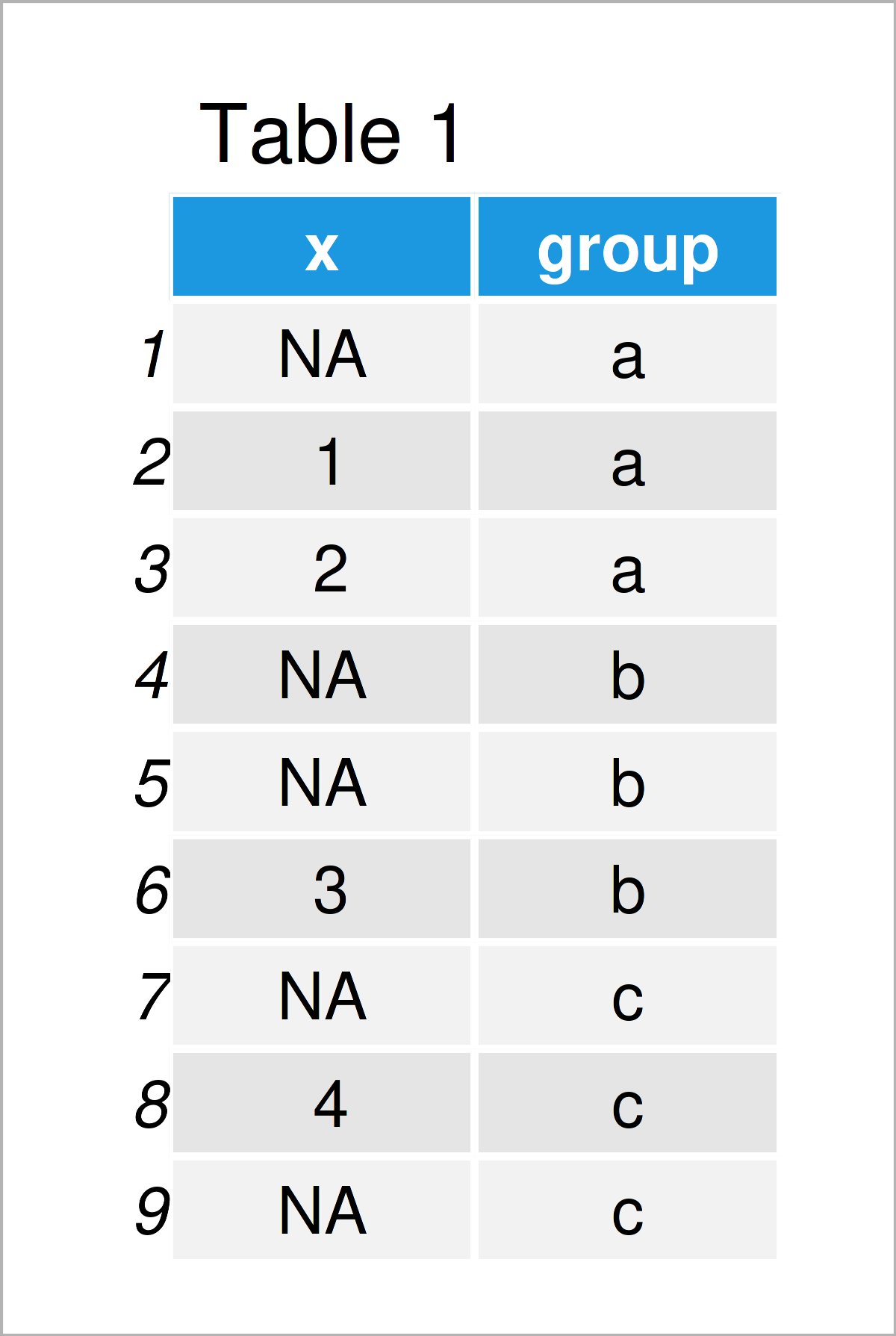# Count NA Values by Group in R (2 Examples)

In this R tutorial you’ll learn how to get the number of missing values by group.

The post will consist of the following content:

## Construction of Example Data

Have a look at the example data below:

```data <- data.frame(x = c(NA, 1, 2, NA, NA, 3, NA, 4, NA),  # Create example data frame
group = rep(letters[1:3], each = 3))
data                                                       # Print example data frame```As you can see based on Table 1, our example data is a data frame and consists of nine rows and two variables. The variable x is numerical and the variable group is a character. The variable x contains several NA values.

## Example 1: Get Number of Missing Values by Group Using aggregate() Function

This example demonstrates how to count the number of NA values by group using the aggregate function of Base R.

Within the aggregate function, we have to specify a user-defined function that counts NA values based on the sum and is.na functions.

Consider the R code below:

```data_count1 <- aggregate(x ~ group,                        # Count NA by group
data,
function(x) { sum(is.na(x)) },
na.action = NULL)
data_count1                                                # Print group counts```Table 2 shows the output of the previous R syntax – We have created a data frame called data_count1 that contains the NA counts by group.

## Example 2: Get Number of Missing Values by Group Using group_by() & summarize() Functions of dplyr Package

In Example 2, I’ll explain how to use the dplyr add-on package to count missing data by group.

In order to use the functions of the dplyr package, we first need to install and load dplyr:

```install.packages("dplyr")                                  # Install dplyr package

Next, we can apply the group_by and summarize functions of the dplyr package to return the number of missing values:

```data_count2 <- data %>%                                    # Count NA by group
group_by(group) %>%
dplyr::summarize(count_na = sum(is.na(x)))
data_count2                                                # Print group counts```In Table 3 it is shown that we have created another count output illustrating the NA values by group.

## Video, Further Resources & Summary

If you need more info on the content of this tutorial, you could have a look at the following video on my YouTube channel. In the video, I’m explaining the examples of this post in R.

Please accept YouTube cookies to play this video. By accepting you will be accessing content from YouTube, a service provided by an external third party.If you accept this notice, your choice will be saved and the page will refresh.

In addition, you might want to read some of the related tutorials that I have published on my website. I have published several articles already:

In this R programming tutorial you have learned how to count the number of NA values by group. In case you have any further comments and/or questions, don’t hesitate to let me know in the comments section below.

Subscribe to the Statistics Globe Newsletter